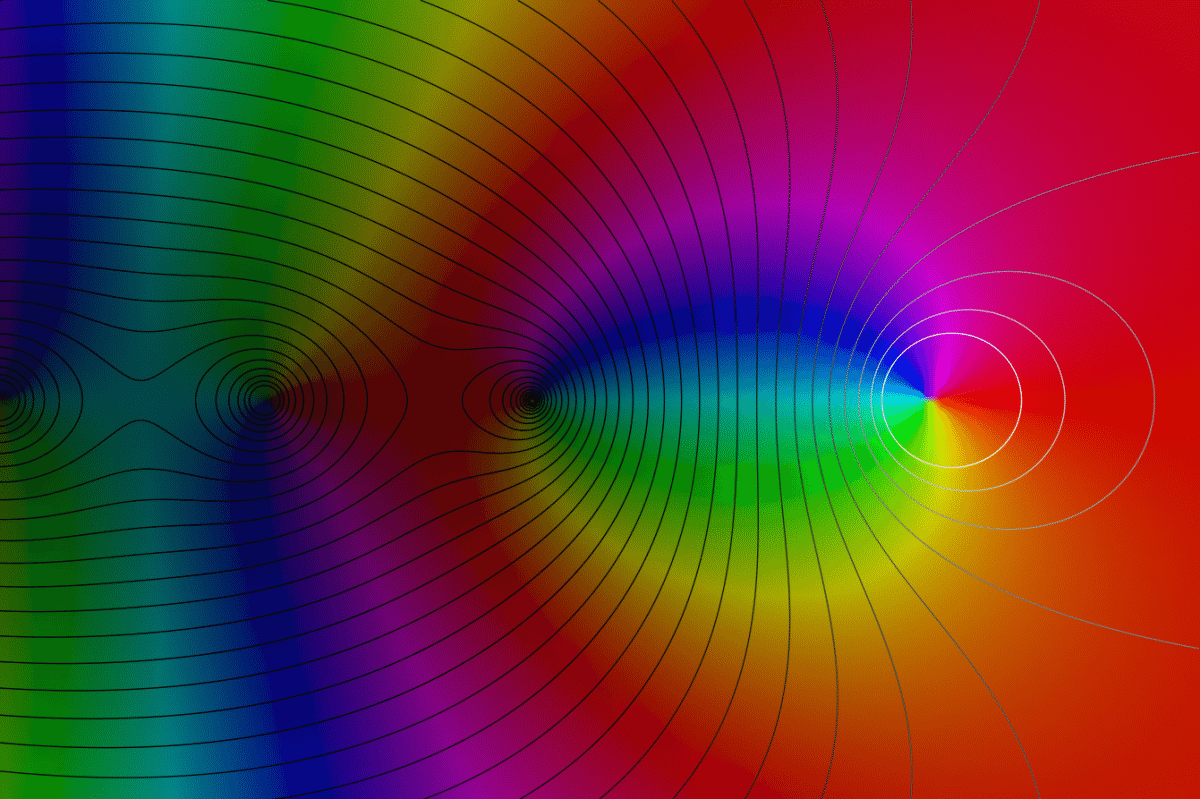# A Slice Of Pi

In Mathematics by Brian Koberlein0 Comments

Today is pi day, which means an obligatory post on that most famous of irrational numbers. Most know the number from geometry, as it was originally defined as the ratio of a circle’s circumference to its diameter. But it turns out there are some deep and subtle connections between pi and physics we still don’t fully understand. Take, for example, the Riemann Zeta Function.

The function is usually denoted as ζ(s), and it looks like a simple sum. Start with the number 1, then add 1/2 to the power of s, then add 1/3 to the power of s, 1/4 to the power of s, and so on. Add that sum forever and you get ζ(s). What does this have to do with pi? Well it turns out that if s is an even number, then our sum will be related to a power of pi. For example, ζ(2) = pi2/6. This is true for any even number, so that ζ(2n) is equal to pi to the 2n power, multiplied by some rational fraction. So it turns out that pi is buried within this infinite series.

But that’s just for the positive even integers. For the negative even integers the sum is simply zero. Thus ζ(-2n) = 0, which are known as the trivial zeros of the Riemann Zeta Function. For ordinary real numbers, the negative even numbers are the only values for which the Riemann Zeta Function is zero. Things get really interesting, however, if we allow for complex numbers.

For any real number multiplied by itself, the answer will always be positive, but there are imaginary numbers that yield a negative number when multiplied by themselves. This are usually denoted by iy, where y is a real number and i represents the square root of -1. Thus the square of i3, for example, is -9. By combining real and imaginary numbers you can get complex numbers, which can be represented by z = x + iy. Complex numbers have a real part x, and an imaginary part iy.

So, if we consider complex numbers, are there any complex zeros where ζ(z) = 0? The answer is yes. In fact we’ve found more than 10 trillion complex zeros, and we know that there are an infinite number of them. What’s interesting about all the known complex zeros is that they can be written as z = 1/2 + iy. In other words, the real part of the number is always 1/2. With trillions of these zeros all having the same form, it would seem clear that all the complex zeros must have their real parts be 1/2. This is known as the Riemann hypothesis, and while it certainly seems to be true, no one has yet been able to prove it absolutely. The Riemann hypothesis is one of the great unsolved mysteries in mathematics.

Complex numbers and circles are related.

It turns out that the Riemann Zeta Function (and thus the Riemann hypothesis) shows up in a wide range of physics theories, from describing the energy levels of certain quantum systems to subtle aspects of string theory. The connection between the Riemann hypothesis and physics is strong enough that some have proposed using experiments with quantum systems as a way to prove the hypothesis (though I doubt that would satisfy mathematicians). There is both deep mathematics and deep physics buried in the Riemann Zeta Function, and we still don’t fully understand it.

Of course all this trouble began when we introduced complex numbers into the function. When I first mentioned complex numbers, I said they could be written as z = x + iy. It turns out they can also be written as a number to an imaginary power. When written that way, complex numbers are multi-valued. That’s because there is a connection between complex numbers and circles. For a circle, if you walk around it you eventually return to your starting point. For complex numbers, increasing the imaginary power is like walking around a circle, so if you add a particular number to the imaginary power you get the same complex number you started with.

That particular number happens to be 2 times (you guessed it) pi.